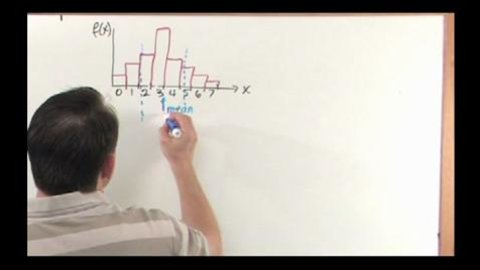# Mean & Standard Deviation of the Binomial Distribution Part of the Series: Probability and Statistics Tutor Series

##### TMW Media## Related videos

Binomial Probability Distribution
This program covers the important topic of the Binomial Probability Distribution in Probability and Statistics. We begin by discussing what the Binomial Distribution is and why it is important. Next, we solve several problems that involve the Binomial Distribution to give students practice with the material.
Standard Deviation and Variance
This program covers the important topic of Standard Deviation and Variance in Probability and Statistics. We begin by discussing what Standard Deviation and Variance is and why it is important. Next, we solve several problems that involve these concepts to give students practice with the material.
Poisson Probability Distribution
This program covers the important topic of The Poisson Probability Distribution in Probability and Statistics. We begin by discussing what the Poisson Distribution is and why it is important. Next, we solve several problems that involve this important distribution to give students practice with the material.
Mean, Median and Mode
This program covers the important topic of Mean, Median, and Mode in Probability and Statistics. We begin by discussing what mean, median, and mode is and why it is important. Next, we solve several problems that involve these concepts to give students practice with the material.
Random Variables and Introduction to Probability Distributions
This program covers the important topic of Random Variables and Probability Distributions in Probability and Statistics. We begin by discussing what a random variable is and how it applies to probability distributions.
Math Series 1
A math series designed for grades 7th to 9th to help students learn and understand math concepts.
Algebra: A Piece of Cake
Covers variable, numerical substitution, algebraic conventions, developing algebraic formulas from number patterns.
Factoring is Fantastic
Key concepts explained: Factoring, terms, expressions, finding the greatest common factor(s), and factoring polynomial expressions that are the sums or differences of terms with common factors. This starts with a review of numerical factors and greatest common factors, and moves into the realm of algebra. Different types of factoring and…
Integer Operations into Negative Zone
Key concepts explained: negative numbers, integers, addition and subtraction of integers.
Linear Equations and Their Graphs
Key concepts explained: linear equations and their graphs, and the domain of an equation.
Percentages: That Make Sense
Part of the Series: Math Series 1
Key concepts explained: converting percentages into decimals and fractions, and converting decimals and fractions into percentages. Taking examples from the widespread use of percentages in everyday life from the percentage of cappuchinos to regular coffee a coffee shop sells, to the percentage of sharks to other fish in an aquarium,…
Slopes: That's a Little bit Steep
Part of the Series: Math Series 1
Key concepts explained: horizontal and vertical lines, positive and negative slopes, calculating a slope by measuring its rise and run, and calculating a slope by using two points on a coordinate plane.